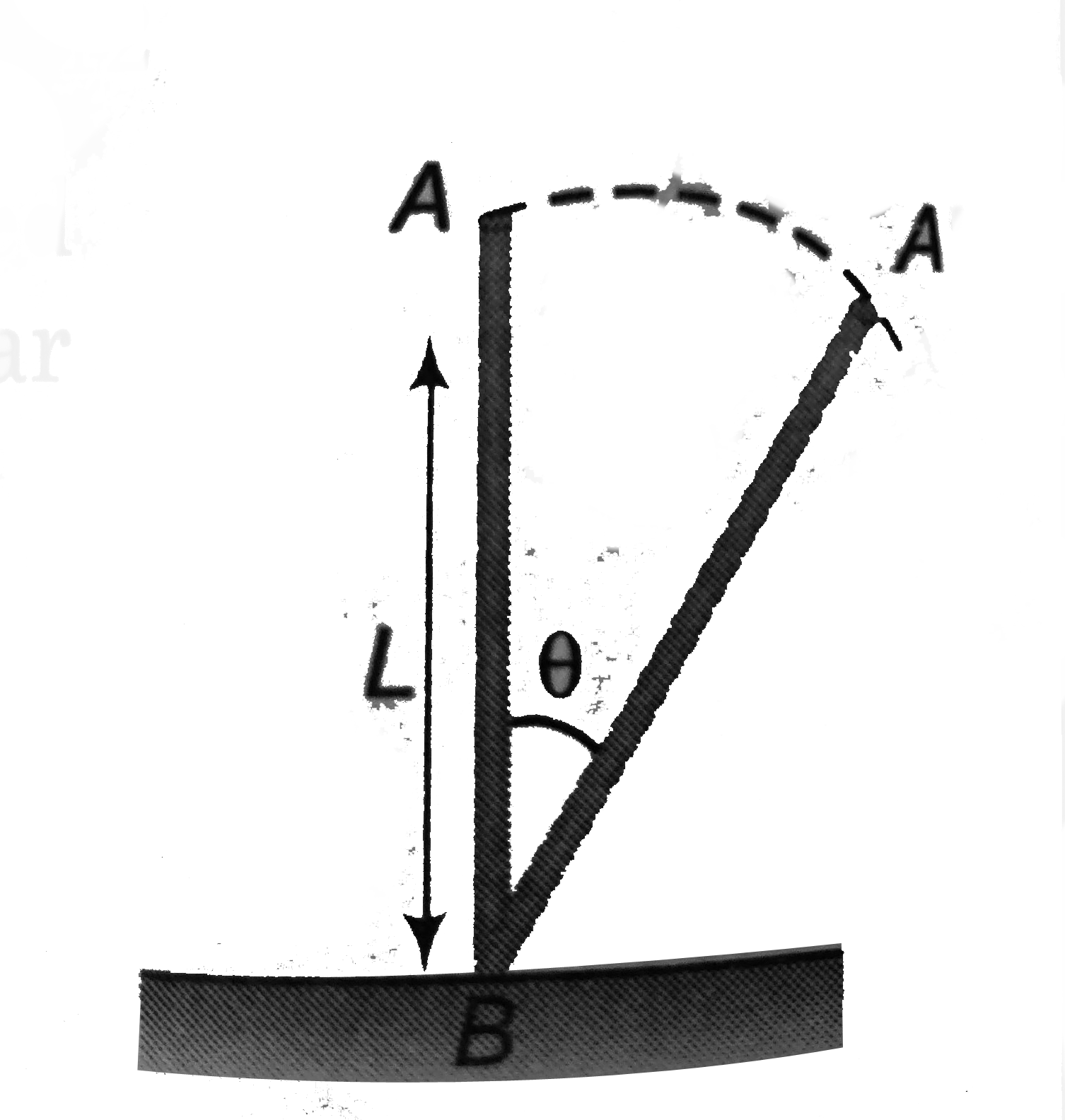# A uniform rod of legth L is free to rotate in a vertica plane about a fixed horizontal axis through B. The rod begins rotating from rest. The angu

36 views
in Physics
closedA uniform rod of legth L is free to rotate in a vertica plane about a fixed horizontal axis through B. The rod begins rotating from rest. The angular velocity omega at angle theta is given as
A. sqrt(((6g)/(L)))sin((theta)/(2))
B. sqrt(((6g)/(L)))cos((theta)/(2))
C. sqrt(((6g)/(L)))sintheta
D. sqrt(((6g)/(L)))costheta

by (75.0k points)
selected

Decrease in gravitational kinetic energy
= increase in rotational kinetic energy
therefore((l)/(2)-(l)/(2)costheta)=(1)/(2)Iomega^(2)
=(1)/(2)((ml^(2))/(3))omega^(2)
omega=sqrt((6g)/(L))sin((theta)/(2))